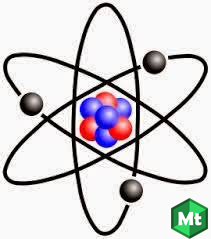# Determine which of each of the following pairs has the higher energy: (a) microwave and infrared; (b) visible and infrared light; (c) ultraviolet light and X-rays; (d) Visible light and ultraviolet light.

Daftar Isi

###-----------------------------------------------------------------------------------------------------------Determine which of each of the following pairs has the higher energy: (a) microwave and infrared; (b) visible and infrared light; (c) ultraviolet light and X-rays; (d) Visible light and ultraviolet light.

(a)    microwave  E = hv

E = (6,626 x 10-34 J s) x (1010 Hz)

E = 6,626 x 10-24 J

Infrared E = hv

E = (6,626 x 10-34 J s) x (1014 Hz)

E = 6,626 x 10-20 J

E microwave + E infrared = 6,626 x 10-24  J +  6,626 x 10-20 J =

0,0006626 x 10-20 J + 6,626 X 10-20 J =

6,626626 X 10-20 J

The higher energy is Infrared light.

(b) visible E = hv

E = (6,626 x 10-34 J s) x (1015 Hz)

E = 6,626 x 10-19 J

Infrared E = hv

E = (6,626 x 10-34 J s) x (1014 Hz)

E = 6,626 x 10-20 J

E visible + E Infrared = 6,626 x 10-19  J + 6,626 x 10-20 J =

6,626 x 10-19 J + 0,6626 x 10-19 J =

7,2886 x 10-19 J

The higher energy is visible light.

(c) ultraviolet E = hv

E = (6,626 x 10-34 J s) x (1016 Hz)

E = 6,626 x 10-18 J

X-rays E = hv

E = (6,626 x 10-34 J s) x (1020 Hz)

E = 6,626 x 10-14 J

E ultraviolet + E X-rays = 6,626 x 10-18 J + 6,626 x 10-14 J =

0,00006626 x 10-14 J + 6,626 x 10-14 J =

6,626 x 10-14 J

The higher energy is X-rays.

(d) visible E = hv

E = (6,626 x 10-34 J s) x (1015 Hz)

E = 6,626 x 10-19 J

ultraviolet E = hv

E = (6,626 x 10-34 J s) x (1016 Hz)

E = 6,626 x 10-18 J

E visible + E ultraviolet = 6,626 x 10-19 J + 6,626 x 10-18 J =

0,6626 x 10-18 J + 6,626 x 10-18 J =

7,2886 x 10-18 J

The higher energy is ultraviolet light.

So, from the above results we can conclude that the greatest energy is in (c), namely ultraviolet light and X-rays, 6,626 x 10-14 J.

Artikel Terkait: